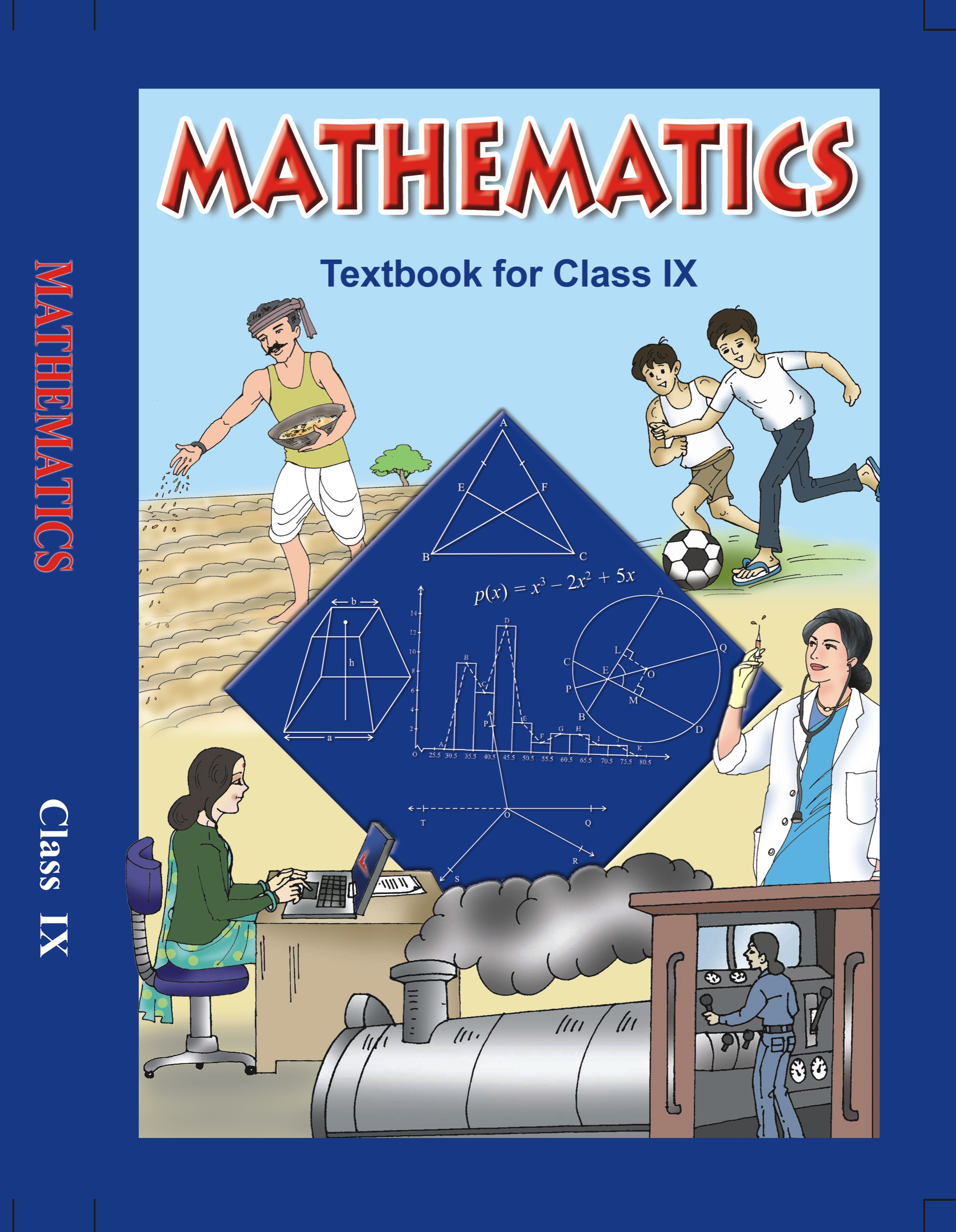# NCERT Solutions for Class 9 Maths Exercise 10.1## myCBSEguide App

CBSE, NCERT, JEE Main, NEET-UG, NDA, Exam Papers, Question Bank, NCERT Solutions, Exemplars, Revision Notes, Free Videos, MCQ Tests & more.

NCERT solutions for Class 9 Maths Circles## NCERT Solutions for Class 9 Mathematics Circles

###### 1. Fill in the blanks:

(i) The centre of a circle lies in _______________ of the circle.

(ii) A point, whose distance from the centre of a circle is greater than its radius lies in _______________ of the circle.

(iii) The longest chord of a circle is a _______________ of the circle.

(iv) An arc is a _______________ when its ends are the ends of a diameter.

(v) Segment of a circle is the region between an arc and _______________ of the circle.

(vi) A circle divides the plane, on which it lies, in _______________ parts.

Ans. (i) Interior

(ii) Exterior

(iii) diameter

(iv) Semi-circle

(v) Chord

(vi) Three

NCERT Solutions for Class 9 Maths Exercise 10.1

###### 2. Write True or False:

(i) Line segment joining the centre to any point on the circle is a radius of the circle.

(ii) A circle has only finite number of equal chords.

(iii) If a circle is divided into three equal arcs each is a major arc.

(iv) A chord, which is twice as long as its radius is a diameter of the circle.

(v) Sector is the region between the chord and its corresponding arc.

(vi) A circle is a plane figure.

Ans. (i) True

(ii) False

(iii) False

(iv) True

(v) False

(vi) True

## NCERT Solutions for Class 9 Maths Exercise 10.1

NCERT Solutions for Class 9 Maths PDF (Download) Free from myCBSEguide app and myCBSEguide website. Ncert solution class 9 Maths includes text book solutions from Mathematics Book. NCERT Solutions for CBSE Class 9 Maths have total 15 chapters. 9 Maths NCERT Solutions in PDF for free Download on our website. Ncert Maths class 9 solutions PDF and Maths ncert class 9 PDF solutions with latest modifications and as per the latest CBSE syllabus are only available in myCBSEguide.

## CBSE app for Class 9

To download NCERT Solutions for Class 9 Maths, Computer Science, Home Science,Hindi ,English, Social Science do check myCBSEguide app or website. myCBSEguide provides sample papers with solution, test papers for chapter-wise practice, NCERT solutions, NCERT Exemplar solutions, quick revision notes for ready reference, CBSE guess papers and CBSE important question papers. Sample Paper all are made available through the best app for CBSE students and myCBSEguide website.